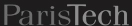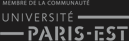Fermer X

# Two Stage Stochastic Optimization for Fixing Energy Reserves

Michel De Lara, Jean-Philippe Chancelier
(last modiﬁcation date: March 18, 2019)
Version pdf de ce document
Version sans bandeaux

### 1 Problem statement

We formalize the problem of ﬁxing energy reserves in a day-ahead market as a two stage stochastic optimization problem. A decision has to be made at night of day J — which quantity of the cheapest energy production units (reserve) to be mobilized — to meet a demand that will materialize at morning of day J + 1. Excess reserves are penalized; demand unsatisﬁed by reserves have to be covered by costly extra units. Hence, there is a trade-oﬀ to be assessed by optimization.

#### 1.1 Stages

There are two stages, represented by the letter t (for time):

• t = 0 corresponds to night of day J;
• t = 1 corresponds to morning of day J + 1.

#### 1.2 Probabilistic model

We suppose that the demand, materialized on the morning of day J + 1, can take a ﬁnite number S of possible values Ds, where s denotes a scenario in the ﬁnite set 𝕊 (S=card(𝕊)).

We denote πs the probability of scenario s, with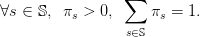(1)

Notice that we do not consider scenarios with zero probability.

#### 1.3 Decision variables

The decision variables are the scalar Q0 and the ﬁnite sequence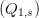s𝕊 of scalars, as follows:

• at stage t = 0, the energy reserve is Q0;
• at stage t = 1, a scenario s materializes and the demand Ds is observed, so that one decides of the recourse quantity Q1,s.

The decision variables can be considered as indexed by a tree with one root (corresponding to the index 0) and as many leafs as scenarios in 𝕊 (each leaf corresponding to the index 1,s): Q0 is attached to the root of the tree, and each Q1,s is attached to a leaf corresponding to s.

#### 1.4 Optimization problem formulation

The balance equation between supply and demand is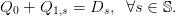(2)

The energies mobilized at stages t = 0 and t = 1 display diﬀerent features:

• at stage t = 0, the energy production has maximal capacity Q0, and producing Q 0 costs 𝒞0(Q0);
• at stage t = 1, the energy production is supposed to be unbounded, and producing Q1 costs 𝒞1(Q1).

We consider the stochastic optimization problem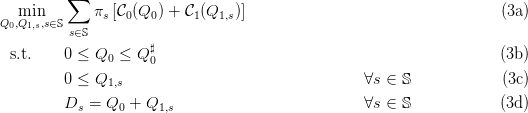Here, we look for energy reserve Q0 and recourse energy Q1,s so that the balance equation (3d) is satisﬁed (at stage t = 1) at minimum expected cost in (3a). By weighing each scenario s with its probability πs, the optimal solution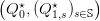performs a compromise between scenarios.

### 2 Formulation on a tree with linear costs

Here, we suppose that the costs are linear: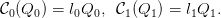(4)

Therefore, the stochastic optimization problem (3) now becomes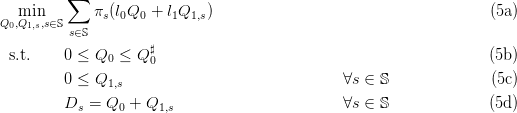This optimization problem (5) is linear. When the number S of scenarios is not too large, we can use linear solvers.

Question 1 We consider the case when 𝕊 = {L,M,H} has S = 3 scenarios (low, medium, high). We want to transform the linear optimization problem (5) under a form adapted to a linear solver.

a)
[1+1+1] Expand the criterion (5a). Expand the inequalities (5b)(5c) into an array of ﬁve scalar equations (one inequality per equation), and the equalities (5d) into an array of three scalar equations (one equality per equation).
b)
[1+1+1+1+1] Let x be the column vector x = (Q0,Q1L,Q1M,Q1H). Propose matrices Ae, Ai and column vectors be, bi and c such that the linear optimization problem (5) can be written under the form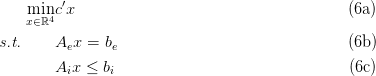Question 2 We are going to numerically solve the linear optimization problem (5).

a)
 Interpret the code below. What is the macro linpro doing? What is lopt? Copy the code into a ﬁle named tp_q1.sce.
b)
[1+2] Solve a numerical version of problem (5) with S = 3 scenarios and the parameters in the code below, by executing the ﬁle tp_q1.sce. What is the optimal value Q0 of the reserve? What is the optimal value Q 1,L? Can you explain why these values are optimal? (there is an economic explanation based on relative costs; there is a mathematical explanation based on the properties of the solutions of a linear program).
c)
 Then, increase and decrease the value of the unitary cost l1 (especially above and below l0). Show the numerical results that you obtain. What happens to the optimal values Q0? Explain why (make the connection with the properties of the solutions of a linear program).

// Formulation on a tree with linear costs.
// Numerical resolution by linear programming

// Constant initialization
S=3;// Number of random scenarios
q0m=30;// max capacity for q0

// Demand
if S==3 then
D=[15;20;50];
Pr=[0.2;0.6;0.2];// Probabilities of Demand
else
D=grand(S,1,'uin',5,50);
Pr=grand(S,1,'unf',0,1);
Pr=Pr ./sum(Pr);// Probabilities of Demand
end

// Constants used in the cost function
ll0=2;ll1=5;

// a revoir pour passer a des contraintes egalité et utiliser lb et ub
c=[ll0;ll1 .*Pr];// cost coefficients

// inequality constraints (bounds on production)
Ai=[-eye(S+1,S+1);eye(1,S+1)];
bi=[zeros(S+1,1);q0m];

// equality constraints, i.e. production equals demand
Ae=[ones(S,1),eye(S,S)];
be=[D];

// solving by linear
// xopt should be [  15,  0, 5, 35 ] when S=3

// scicoslab version with linpro
A=[Ae;Ai];b=[be;bi];
[xopt,lopt,fopt]=linpro(c,A,b,[],[],size(be,'*'))

Question 3 We are going study the impact of the number S of scenarios on the numerical resolution of the linear optimization problem (5).

a)
 Take S = 100. What is the optimal value Q0 of the reserve? Identify the scenario s with the lowest demand. What is the optimal value Q1,s? Explain.
b)
 For what value of n in S = 10n can you no longer solve numerically?

### 3 Formulation on a tree with quadratic convex costs

Here, we suppose that the costs are quadratic and convex: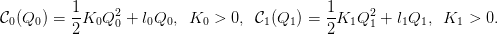(7)

The optimization problem (3) is quadratic convex: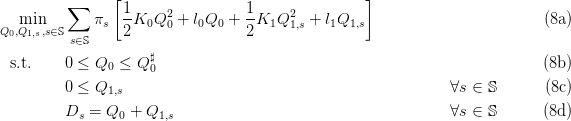When the number S of scenarios is not too large, we can use quadratic solvers.

Question 4 We are going to numerically solve the quadratic convex optimization problem (8).

a)
 Interpret the code below. What is the macro quapro doing? Copy the code into a ﬁle named tp_q2.sce.
b)
 Solve a numerical version with S = 3 scenarios and the parameters in the code below, by executing the ﬁles tp_q1.sce and tp_q2.sce. What is the optimal value Q0 of the reserve? What is the optimal value Q1,L?
c)
[1+1+2] Check numerically that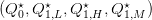is an inner solution to the optimization problem (8a), that is, check numerically that the inequalities (8b) and (8c) are strict. What is the diﬀerence with the optimal solution of Question 2? Discuss the diﬀerence (make the connection with the properties of the solutions of a linear program).
d)
[2+1] Compute the derivatives of the cost functions in (7). Check numerically (giving the details of computation) that the optimal solution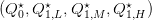satisﬁes the following relation between marginal costs: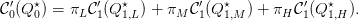(9)

// Formulation on a tree with quadratic costs
// Numerical resolution by brute force quadratic programming

// just to get common data
exec('tp_q1.sce');

A=[Ae;Ai];b=[be;bi];
KK0=10;KK1=1;
KK=diag([KK0,KK1*Pr' .*ones(1,S)]);

// scicoslab version with quapro
[xopt,lopt,fopt]=quapro(KK,c,A,b,[],[],size(be,'*'))

Question 5 We are going to numerically solve the quadratic convex optimization problem (8) after changing the relative values of the (quadratic) parameters K0 and K1. For the parameters K0, l0, l1, we take the same values as those in Question 2 (hence l1 > l0) and in Question 4.

a)
 Take K1 > K0 with K1 K0. What are the optimal value Q0 of the reserve and the optimal value Q1,L?
b)
 Take K1 > K0 with K1 >> K0. What are the optimal value Q0 of the reserve and the optimal value Q1,L?
c)
 Discuss.

Question 6 We are going study the impact of the number S of scenarios on the numerical resolution of the quadratic convex optimization problem (8).

a)
 Take S = 100. Solve a numerical version of problem (8) with the parameters K0, l0, K1, l1 as in the code of Question 4. What is the optimal value Q0 of the reserve? Identify the scenario s with the lowest demand. What is the optimal value Q1,s?
b)
 For what value of n in S = 10n can you no longer solve numerically?

Question 7 This theoretical question may be ignored
(by those who want to focus on numerical results)
For this question, we temporarily ignore the inequalities (8b) and (8c) in (8). Therefore, we consider the optimization problem (8a) with equality constraints (8d), that is: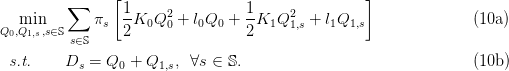a)
 Compute the Hessian matrix of the criterion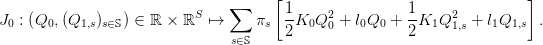(11)

What are the dimensions of the Hessian matrix?

b)
 Why does optimization problem (10) have a solution? (Beware of the domain)
c)
 Why is the solution unique?
d)
[1+2] Why are the equality constraints (10b) qualiﬁed? Why does an optimal solution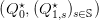of (10) satisfy the Karush-Kuhn-Tucker (KKT) conditions (ﬁrst-order optimality conditions)?
e)
 Why is a solution of the KKT conditions an optimal solution of (10)?
f)
 Write the Lagrangian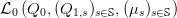associated with problem (10).
g)
 Deduce the KKT conditions. Show that there exist (μs,s 𝕊) such that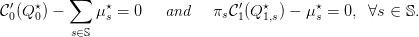(12)

h)
 Deduce that — whenis an inner optimal solution to problem (8a) we have the following relation between marginal costs: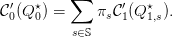(13)

Give an economic interpretation of this equality.

### 4 Formulation on a fan with quadratic convex costs

When the number S of scenarios is too large, Problem (5) — be it linear or convex — cannot be solved by direct methods.

#### 4.1 Dualization of non-anticipativity constraints

To bypass this problem, we use a “trick” consisting in introducing new decision variables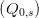s𝕊, instead of the single decision variable Q0, and we write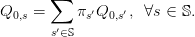(14)

These equalities are called the non-anticipativity constraints. Indeed, the equations (14) express that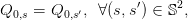(15)

that is, the decision at stage t = 0 does not depend on the scenario s, hence cannot anticipate the future. Later, we will treat the constraints (14) by duality.

Therefore, the stochastic optimization problem (3) now becomes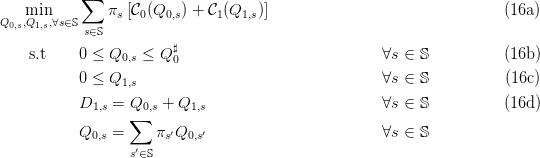By the assumption (1) that there are no scenarios with zero probability (πs > 0), we replace each equality in the last equation by the equivalent one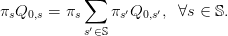(16e)

We attach, to each equality above a multiplier λ0,s. We put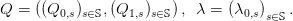(17)

The corresponding Lagrangian is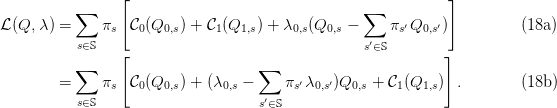Question 8 This theoretical question may be ignored
(by those who want to focus on numerical results)
When the costs are quadratic and convex as in (7), show that

a)
 the criterion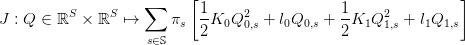(19)

in (16a) is a-strongly convex in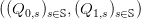, and provide a possible a > 0,

b)
 the domain deﬁned by the constraints in the optimization problem (16) is closed,
c)
 the domain deﬁned by the constraints in the optimization problem (16) is convex,
d)
[2+1] the optimization problem (16) has a solution, and it is unique, denoted by Q,
e)
 there exists a multiplier λ such that (Q) is a saddle point of the Lagrangian  in (18).

#### 4.2 Uzawa algorithm

We consider the following optimization problem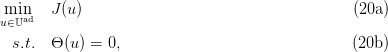under the assumptions that

• the set 𝕌ad is a closed convex subset of a Euclidian space N,
• the criterion J : N is an a-strongly convex (a > 0) and diﬀerentiable function,
• the constraint mapping 𝜃 : N M is aﬃne, κ-Lipschitz (κ > 0),

Then the following algorithm — called dual gradient algorithm, or Uzawa algorithm — converges toward the optimal solution of Problem (20), when 0 < ρ < 2a∕κ2.

Data: Initial multiplier λ(0), step ρ Result: optimal decision and multiplier; repeat u(k) = arg min u𝕌ad(u,λ(k)) ;
λ(k+1) = λ(k) + ρΘ(u(k)) ; until Θ(u(k)) = 0;

#### 4.3 Numerical resolution by Uzawa algorithm (quadratic convex case)

When the costs are quadratic and convex as in (7), the optimization problem (16) becomes: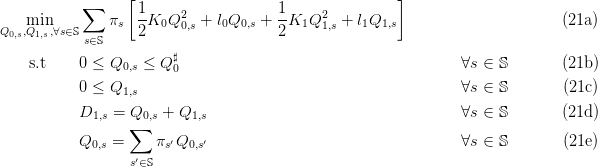Question 9 This theoretical question may be ignored
(by those who want to focus on numerical results)
When the costs are quadratic and convex as in (7), identify in the optimization problem (21) the corresponding elements in the Uzawa algorithm .1:

a)
 decision variable u,
b)
 decision set N (for what N?),
c)
 aﬃne constraints mapping Θ : N M, corresponding to the constraints (21e) (why is it κ-Lipschitz, and for which κ?).
d)
 Explain why the Uzawa algorithm converges towards the optimal solution of Problem (21).

Question 10 We are going to numerically solve the quadratic convex optimization problem (21) by Uzawa algorithm.

a)
 Detail what the code below is doing ; explain how the code implements the Uzawa algorithm. Why do we use the macro quapro? What is the dual function? Copy the code into a ﬁle named tp_q3.sce.
b)
 Solve a numerical version with S = 3 scenarios. What is the solution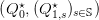given by the algorithm? Do you have that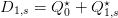, for all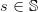?
c)
 Then, try with S = 100. What is the value Q0 of the reserve given by the algorithm? Identify the scenario s with the lowest demand. What is the value Q1,s given by the algorithm?
d)
 For what value of n in S = 10n can you no longer solve numerically?

// formulation on a fan
// Constant initialization

// Constant initialization
S=3;// Number of random scenarios
q0m=30;// max capacity for q0

// Demand
if S==3 then
D=[15;20;50];
Pr=[0.2;0.6;0.2];// Probabilities of Demand
else
D=grand(S,1,'uin',5,50);
Pr=grand(S,1,'unf',0,1);
Pr=Pr ./sum(Pr);// Probabilities of Demand
end

// Constants used in the cost function
ll0=2;ll1=5;
KK0=10;KK1=1;

// Uzawa iterations when the dualized constraints are the S constraints
//  Q0(ss) = sum(Pr.*Q0);

Q0=zeros(S,1);
Q1=zeros(S,1);
f0=zeros(S,1);

rho=5;

lambda=zeros(S,1);
// iterations of the Uzawa algorithm
for it=0:30 do
// decomposed minimizations (loop over scenarios ss)
for ss=1:S do
// inequality constraints (bounds)
// bounds on (Q0s,Q1s)
ll=[ll0*Pr(ss);ll1*Pr(ss)];// cost coefficients
Ai=[-eye(2,2);eye(1,2)];
bi=[zeros(2,1);q0m];
// equality constraints i.e production equals demand
Ae=[1,1];
be=[D(ss)];
A=[Ae;Ai];b=[be;bi];
KK=Pr(ss)*diag([KK0,KK1]);
//
cc=ll+Pr(ss)*[lambda(ss)-sum(Pr .*lambda);0];
[xopt,lopt,fopt]=quapro(KK,cc,A,b,[],[],size(be,'*'));
Q0(ss)=xopt(1);
Q1(ss)=xopt(2);
f0(ss)=fopt;
printf("Dual function %f\n",sum(f0));
end
Q0bar=sum(Pr .*Q0);
lambda=lambda+rho*(Pr .*(Q0-Q0bar));
end

### 5 Formulation on a fan with linear costs

Here, we suppose that the costs are linear, as in (4).

#### 5.1 Diﬃculties in applying Uzawa algorithm with linear costs

The optimization problem (16) becomes: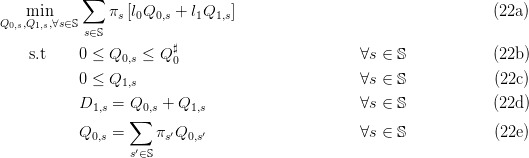Question 11 We are going to numerically solve the linear optimization problem (22).

a)
 Detail what the code below is doing. Why do we use the macro linpro? Copy the code into a ﬁle named tp_q4.sce.
b)
 Solve a numerical version with S = 3 scenarios. What do you observe regarding convergence of the Uzawa algorithm? Can you explain why?

// formulation on a fan with linear cost
// Uzawa does not work

S=3;// Number of random scenarios
q0m=30;// max capacity for q0
D=[15;20;50];
Pr=[0.2;0.6;0.2];// Probabilities of Demand
rho=0.1;
// Constants used in the cost function
ll0=2;ll1=5;

// Uzawa iterations when the dualized constraints are the S constraints
//  Q0(ss) = sum(Pr.*Q0);

Q0=zeros(S,1);
Q1=zeros(S,1);
f0=zeros(S,1);

lambda=zeros(S,1);
for it=0:30 do
// decomposed minimizations
for ss=1:S do
// inequality constraints (bounds)
// bounds on (Q0s,Q1s)
ll=[ll0*Pr(ss);ll1*Pr(ss)];// cost coefficients
Ai=[-eye(2,2);eye(1,2)];
bi=[zeros(2,1);q0m];
// equality constraints i.e production equals demand
Ae=[1,1];
be=[D(ss)];
A=[Ae;Ai];b=[be;bi];
//
cc=ll+Pr(ss)*[lambda(ss)-sum(Pr .*lambda);0];
[xopt,lopt,fopt]=linpro(cc,A,b,[],[],size(be,'*'));
Q0(ss)=xopt(1);
Q1(ss)=xopt(2);
f0(ss)=fopt;
printf("Dual function %f\n",sum(f0));
end
Q0bar=sum(Pr .*Q0);
lambda=lambda+rho*(Pr .*(Q0-Q0bar));
end

#### 5.2 Dualization of non-anticipativity constraints

To bypass the diﬃculty in applying Uzawa algorithm with linear costs, we use a “trick” consisting in introducing new decision variables Q0 ands𝕊, instead of the single decision variable Q0, and we write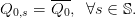(23)

These equalities are another form of the non-anticipativity constraints. Indeed, the equations (23) express that the decision at stage t = 0 cannot anticipate the future, hence cannot depend on the scenario s. We will treat these constraints by duality.

Therefore, the stochastic optimization problem (3) now becomes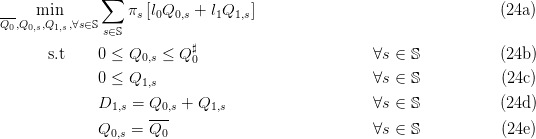By the assumption (1) that there are no scenarios with zero probability (πs > 0), we replace each equality in (24e) by the equivalent one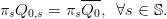(25)

We attach, to each equality above a multiplier λ0,s. We put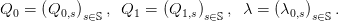(26)

#### 5.4 Progressive Hedging algorithm (quadratic solver)

Question 12 We are going to numerically solve the linear optimization problem (24) by the Progressive Hedging algorithm.

a)
 Detail what the code below is doing. Why do we use the macro quapro? Explain the two roles of the new parameter rr. Copy the code into a ﬁle named tp_q5.sce.
b)
 Solve a numerical version with S = 3 scenarios. What do you observe regarding convergence of the Uzawa algorithm? Can you explain why?

// formulation on a fan
// with linear cost and augmented lagrangian

S=3;// Number of random scenarios
q0m=30;// max capacity for q0
D=[15;20;50];
Pr=[0.2;0.6;0.2];// Probabilities of Demand
// Constants used in the cost function
ll0=2;ll1=5;
// Constant used both as a quadratic term and as a gradient step
rr=0.1;

// Uzawa iterations when the dualized constraints are the S constraints
//   Q0s = sum(Pr.*Q0)

Q0=zeros(S,1);
Q1=zeros(S,1);
f0=zeros(S,1);

lambda=zeros(S,1);
Q0bar=0;
for it=0:300 do
// decomposed minimizations
// we alternate minimization
for ss=1:S do
// inequality constraints (bounds)
// bounds on (Q0s,Q1s)
ll=[ll0*Pr(ss);ll1*Pr(ss)];// cost coefficients
Ai=[-eye(2,2);eye(1,2)];
bi=[zeros(2,1);q0m];
// equality constraints i.e production equals demand
Ae=[1,1];
be=[D(ss)];
A=[Ae;Ai];b=[be;bi];
KK=rr*Pr(ss)*diag([1,0]);
cc=ll+[Pr(ss)*lambda(ss);0];
cc=cc+[-rr*Q0bar*Pr(ss)];
[xopt,lopt,fopt]=quapro(KK,cc,A,b,[],[],size(be,'*'));
Q0(ss)=xopt(1);
Q1(ss)=xopt(2);
f0(ss)=fopt;
end
Q0bar=sum(Pr .*Q0);
lambda=lambda+rr*(Pr .*(Q0-Q0bar));
end

// solution (Q0bar,Q1)
Q1=max(0,(D-Q0bar));
// to be compared with tp_q1

### A Additional code for “Formulation on a tree with linear costs”

// Formulation on a tree with linear costs.
// Numerical resolution by linear programming
// using nsp linprog (glpk).

// Constant initialization
S=3;// Number of random scenarios
q0m=30;// max capacity for q0

// Demand
if S==3 then
D=[15;20;50];
Pr=[0.2;0.6;0.2];// Probabilities of Demand
else
D=grand(S,1,'uin',5,50);
Pr=grand(S,1,'unf',0,1);
Pr=Pr ./sum(Pr);// Probabilities of Demand
end

// Constants used in the cost function
ll0=2;ll1=5;

// a revoir pour passer a des contraintes egalité et utiliser lb et ub
c=[ll0;ll1 .*Pr];// cost coefficients

// inequality constraints (bounds on production)
Ai=[-eye(S+1,S+1);eye(1,S+1)];
bi=[zeros(S+1,1);q0m];

// equality constraints, i.e. production equals demand
Ae=[ones(S,1),eye(S,S)];
be=[D];

// solving by linear
// xopt should be [  15,  0, 5, 35 ] when S=3

if exists('%nsp') then
// in nsp linprog is glpk
[xopt,fopt,flag]=linprog(c,Ai,bi,Ae,be);
// we can also use quapro if available with a 0 quadratic term
if exists('quapro','callable') then
A=[Ae;Ai];b=[be;bi];
[xopt_q,lagr_q,fopt_q]=quapro(zeros(S+1,S+1),c,A,b,[],[],size(be,'*'))
end
else
// scicoslab version with linpro
A=[Ae;Ai];b=[be;bi];
[xopt,lopt,fopt]=linpro(c,A,b,[],[],size(be,'*'))
end

### B Additional code for “Formulation on a tree with quadratic convex costs”

// Formulation on a tree with quadratic costs
// Numerical resolution by brute force quadratic programming
// using nsp quapro or optim

if exists('%nsp') then
end

// just to get common data
exec('tp_q1.sce');

// the problem is now quadratic; we use a quadratic solver if available
A=[Ae;Ai];b=[be;bi];
KK0=10;KK1=1;
KK=diag([KK0,KK1*Pr' .*ones(1,S)]);

if exists('%nsp') then
// we can also use quapro if available with a 0 quadratic term
if exists('quapro','callable') then
[xopt_q,lagr_q,fopt_q]=quapro(KK,c,A,b,[],[],size(be,'*'))
end
else
// scicoslab version with quapro
[xopt,lopt,fopt]=quapro(KK,c,A,b,[],[],size(be,'*'))
end

// BEWARE: using a global optim if quapro is not available
// we eliminate the equality constraint to use optim on q0

function [f,g,ind]=costf(q0,ind)
q1=max(0,(D-q0));
// expression of the cost
f=sum(Pr .*(ll0*q0+ll1*q1))+(1/2)*[q0;q1]'*KK*[q0;q1]
// expression of the cost derivative
g=sum(Pr .*(ll0-ll1*(D-q0 >= 0)))+KK(1,1)*q0+-sum((D-q0 >= 0) .*(KK(2:\$,2:\$)*q1))
endfunction

if exists('%nsp') then
Q0=optim(costf,0,xinf = 0,xsup = q0m)
else
[fo,Q0]=optim(costf,'b',0,q0m,0)
end

// solution (Q0,Q1)
Q1=max(0,(D-Q0))
 L'École des Ponts ParisTech est membre fondateur de L'École des Ponts ParisTech est certifiée